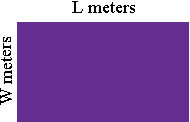SEARCH HOMEMath Central Quandaries & QueriesQuestion from marylyne, a student: the perimeter of the rectangular is 70 meters. if the width is 40% of the length, find the dimensions?Marylyne,

Suppose the length is L meters and the width is W meters.The perimeter is then L + W + L + W = 2L + 2W meters.

Since W is 40% of L, W = 0.40 × L.

Hence you have three facts.

• The perimeter is 70 meters.

• The perimeter is 2L + 2W meters.

• W = 0.40 × L.

Put these three facts together to form an equation in the variable L. Solve this equation for L.

PennyMath Central is supported by the University of Regina and The Pacific Institute for the Mathematical Sciences.